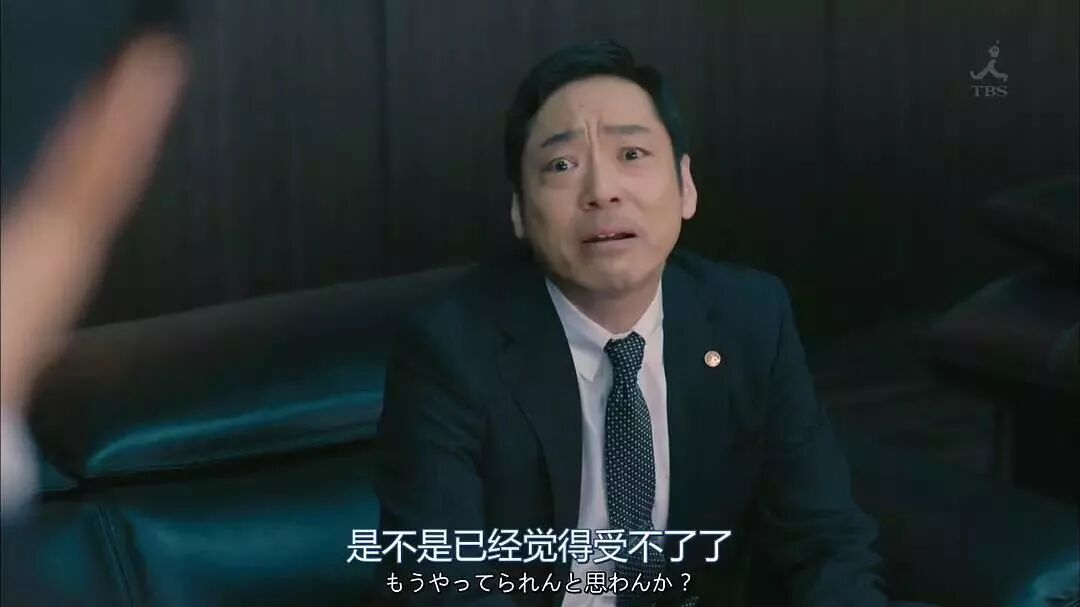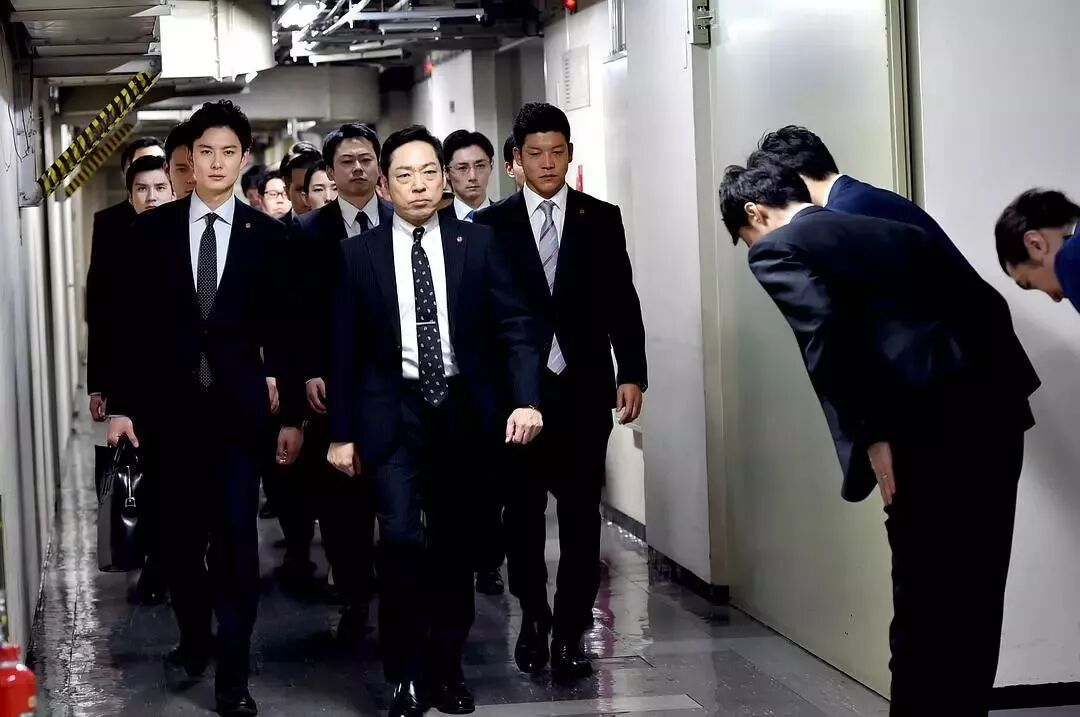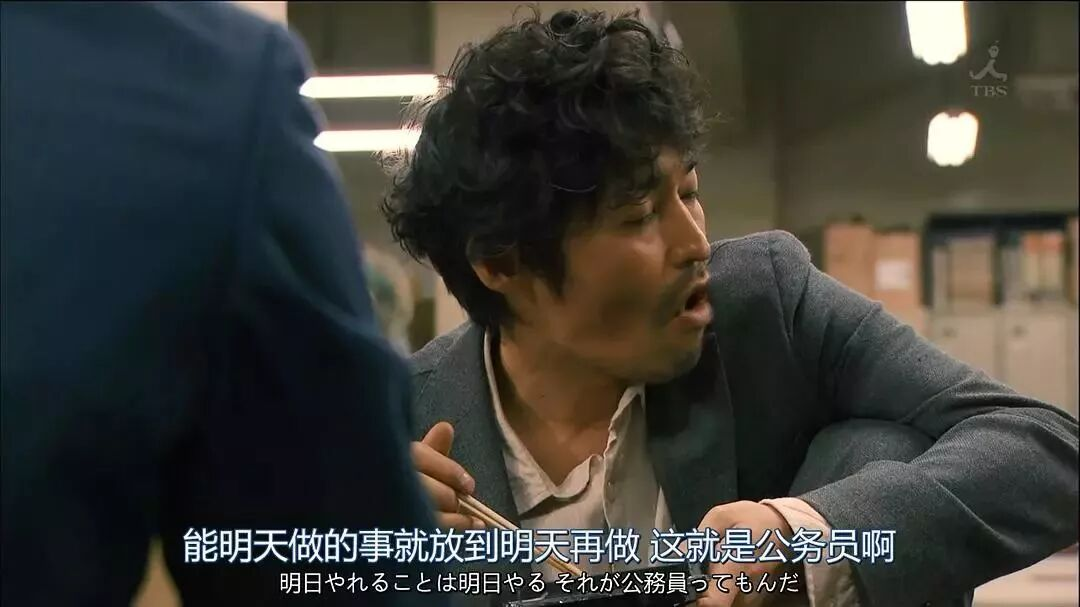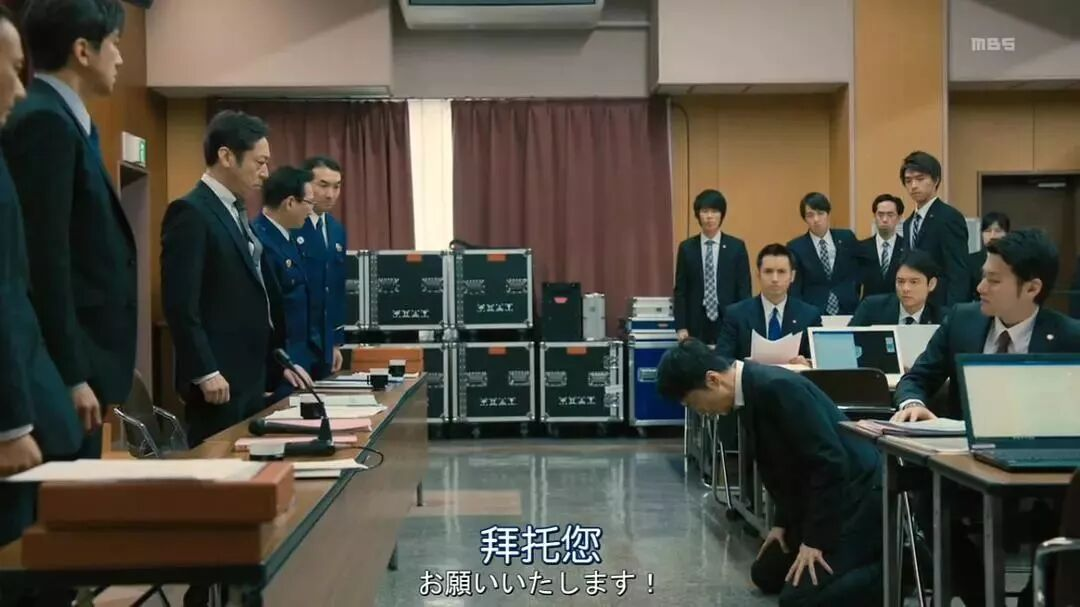## [同事亲友] 那些离开体制内的年轻人||真实故事计划 麦子熟了

### 马上注册，结交更多好友，享用更多功能，让你轻松玩转社区。

x9 s& k# ~: b2 w- G8 `5 t

" ^8 v4 p0 }2 k/ ~( N8 Z  U  b- e

+ [0 S. e% W7 s9 {- k3 }" [& H

; [2 f, i  z3 c! C

) d: i( @4 }7 u/ L5 o+ |5 q4 }" H
* ^0 e- I* b. u8 j5 C

* c0 M/ H0 l" Q" u7 k

6 Q. I- M# o' t* Q

1月25日，国考笔试出分。又有一批年轻人即将进入体制内，拿起铁饭碗。

9 n\$ y. Q; k7 i6 Y

" o' O! c6 w# u8 ^6 u

2 L" ~/ }/ U. A; L8 m1 s* P& }: g6 }0 _

8 W# w3 a+ Y) z' ^3 D

4 @( V+ j5 H' V3 Q. R

2 H% m1 B" q2 r- \7 {! g; J

Part
1

! p% s* c) i# J+ i) Y6 m! q

& G5 i' _8 U: S+ X\$ J( q5 e; ~! `

" ?* m1 ^/ `. j* [& i& Q

) \& E1 `& w  b  @

6 w5 G9 a- y9 q# \' L1 |\$ n
1 M% y/ j3 S% T

“跳车！跳啊！快他妈跳啊！”

7 L\$ L5 ^5 ^. a1 X0 d+ |+ I

& ~+ Y+ x5 S5 H! x' o

# b( z% d6 p% w( f6 t  A! D! ?+ }+ e; W6 b) A\$ ~5 d

; A# L/ h# w% V/ P& V/ P. t( h

/ r4 p; D3 S, d5 A0 y

4 }6 j' K3 n9 L: l! x1 r& }; x0 i

7 [  }7 V! @: v5 _* Q

. {2 S; s% e% i" Q- I
1 U9 ^  ^8 u" e: \0 L( V+ d  w* @1 P  {' r) W6 ^. Y/ p

0 j2 ]9 u5 o: O' d. N# n) f/ @

, Z, e) `; @7 l5 c/ g8 a/ g

E7 L1 R  @( w+ l# v  t\$ K) K

2 n1 G! [. a) k( I4 A\$ b0 S
! A. a) P7 q3 H6 y* |6 K

0 F' d# U# t8 k  u7 V. g% g& o2 H: ~
. F\$ ~) M; I! q0 N0 ^7 S

% F. [, N" v) |2 `# Q" E7 @, E% W. J: `3 C  [

0 O1 }, w- f' f( W) Z/ J
' r" K\$ k# t0 ~2 o4 X# ?  q

8 y: t; f1 `8 }/ h4 e: H

; q7 _* L6 K* j3 Y& H& B! V* S

* y! a8 G9 B) A\$ g% b* N
; f2 _( e* w7 ^' S4 ^8 Z; q0 `) R. T" T& [4 e/ `

, V! Z# A" [* N- `7 o+ p
\$ Q6 B6 }( {7 j- _. Y+ f/ F

# C3 c8 y% g\$ p9 X
Part
2
+ q. V9 ^+ x3 [' Z0 K

8 A, G0 Y" L5 i! m7 S3 F

% v' O5 R3 M& g
\$ R0 F1 @( E0 M
2010年，我拿到了大专自考本科的文凭，当时伯伯是烟草局里的二把手，给弄了个名额，我就开始在市里的烟草局上班。
' ?5 E: x8 s/ m0 y. }! N9 B! l9 w
1 ]- u& I0 Y% L8 E# |

# ^' B" Z  {9 {\$ E+ w

6 a9 E5 p2 D: k7 \
“我刚从后面看，还以为哪个大叔走错包间了。”

+ s6 N( u( [6 Z; w2 b/ K\$ n

; \; Q+ \! x' F- F/ i; A

) T. r4 G0 B5 Y, }/ w# |
G- r* f5 i- j: d% K7 C3 m\$ A1 v- w3 J8 ~2 n! M\$ o

4 l! z/ E  a. ~+ \0 m) A) |" g& [( G

1 n  N; f* J! e( E( x" h

% n  z0 l& v) F' X3 E" r\$ O! \

% @8 D/ R+ H) ^; M

, _1 [  I1 Q, {( j- s" q2 k\$ m% ?

6 j, I% O. K, Y( E! T5 D, X% j
“以前都是我错了，我余生给你做牛做马，你不要糟蹋自己。”

- l  H& {& s+ `2 S) s/ ]1 O

4 y. a( V0 {0 o\$ z: w9 G! C/ U  R' ]6 c; [/ k
1 H% k9 u7 S& N; P, ^

2 V5 b2 y3 e* t
“冤枉了就冤枉了，老子就是要冤枉他。”

! T# I! @/ T' U. j

6 u. J; `: l5 O1 v0 z

/ {\$ X8 B\$ f  H; y

\$ ]4 Y0 K! H( L+ y0 s\$ G& O
- d% ^( o. b2 A

0 d( g' w+ u1 x" g. E2 B4 }, o% V

f5 P' J% i, r# }8 p\$ g/ \

) R, l" [2 P0 F1 Y
9 v; r" p" @3 l

- X" Q0 v8 k) R( y

. |- m1 L8 M5 [6 Y: u* |- \3 z  Q, u

- [' E2 p\$ K8 r0 p+ m

, s) S4 c/ ?' \8 Y# W

6 ^9 |/ o. Y- r# _8 n( [2 S' U5 [

! w: i9 n9 i* Q! n9 f

. E; g: }0 e* r+ a
, X& {\$ o- L. W% ~6 r; U/ R" J\$ e8 x; n) E
' C" f* c4 z5 S5 ?2 H4 r2 H

3 o; v/ k% s1 ~% G: }

/ ?- |; N4 @- B; P/ d

3 p( s8 K- H! k8 u
7 z, [, A* r4 o8 @

, {' ?; }4 x- J1 j
3 H1 C  W  L\$ x/ y( f4 K' C3 ?: E& }3 ^1 q

. a% d8 w4 s& H( D) S

# e) \+ o" y' S: k\$ h* S+ ~

( g! c* R  h: a& R, p1 d8 ^4 _
# N8 U% i; F5 E, n6 G& Y

" p1 K+ d- |1 j# O4 \5 x

* R) i9 I0 U1 p% w9 @# A
5 n  Z( k! s. H. X9 _: @

; h* l- J4 f8 |4 T1 l2 b9 ]

9 N# ^& z3 R\$ E: h, Z

% j* f# c  l, l- H, l* h\$ |

: p: i# G# L' F\$ w

7 U- i/ A3 u9 ]% o" F, C- H

2017年，从年初十到五一，他每天早上七点出门，晚上十二点回家，没有机会说话。
8 x9 j& Q" u4 w) }- G

7 [, b# B" h- n- [0 F

. z2 `\$ ~- [" s\$ D# A7 `
% V  v/ T  e2 n& B- r2 U- R+ y4 }, L! j/ [

' D6 p, \* W1 G) z1 h9 {

8 @3 J1 H, P" E* N! N

) s) o0 u& V. J# I# X+ v
2 n. W( V/ S7 U  M

9 A4 W4 j) P; g3 ?7 V

G1 {8 ^2 N! {

“我们是副股级后备干部。”
3 P4 M! l! K\$ {+ \+ J
4 @* m% B# Y+ W2 x. Z3 Q8 k: b

4 ]2 G; r( R" r; x2 ^  ?: g  R

% ^% f3 Z  U7 W4 l( W, G

m- V+ [9 A) b% f/ b\$ P, M" Q% c7 h5 T" o! ]+ m
. A' Y\$ U  T, f+ n\$ x\$ r
2014年中期，媒体行业的不景气已经扩散开来，很多同事都选择了辞职，加入大的互联网公司，甚至是自己创业。

4 u7 m7 I: o\$ [1 r+ x6 w; f

5 r  G6 e4 w! C4 k
! o  I% m; P) _2 R: H

. {) o8 J/ F" C, ]\$ i1 O

) K- C# h) f+ m; u+ _

/ v3 B) }& V' F+ ]\$ [8 I5 l0 [' V

- E. T5 E1 v( r5 V" O) L2 E5 b* Q7 V1 i8 c3 W, \; i

]7 ]( g0 a/ }  _
“我们只能谈这些很俗的事情了。”

/ I\$ Z\$ G8 u\$ {# w/ _6 H7 ?

8 i+ d5 |3 h) ~9 j1 a3 i/ U

1 j. ]/ x: r+ J1 i2 f

. `( U; m! O" U7 p9 _! s

^; z* e, s/ I. R
) k\$ L, V* M% x+ [6 \
# _: N0 L\$ p6 \' z# m9 Y

( q0 p. r* x\$ T- Q1 H; F
Part
3

1 p& R! l+ `* d. r\$ x  s/ z
; u6 f4 n! N( M0 }, ~' R9 H  L. e

. o& Q' Q! M7 W7 f

! d# }2 z  x7 q1 [- E
% S( L% b6 v6 y+ ?! o& d' Q. X! J

5 p* Q) q! w- E, P+ ^

7 q# N. ^6 [% y6 N: x# Y
2 H8 t1 q2 k7 A. E7 n

" r  a& S2 M; |: e, H' U1 p

5 ~# G0 p% p7 K7 B# h
/ {9 C1 C9 F6 R8 g\$ ?5 [/ f. e" B+ K, k: r

2 X" U# g4 ^, {1 c1 S8 F

! `! \+ G, S  `. M+ }( N
. L6 y2 z, T! b* Z; U# V) ~

* u) f" l' e0 R' p( V

- m  j+ ]\$ S' E

' z: k* r: l  B' x
# ?- e0 \/ S- T\$ M4 F3 F

6 g- ]3 }' `6 l! p7 W( l5 W
/ W, }/ _\$ d. r# c0 G8 Z

" W- B7 A9 j4 l5 j5 `

0 c8 c/ f: H: ]6 G+ {/ v

, C; p/ p\$ d1 U5 u. B

1 m8 v\$ q  |& M; c( y
; i5 |' T, d3 L/ \4 G/ ]

! U: A! J7 `( Q% x

\$ i9 a+ c" ]* K! G6 ^
# \! w' a3 b; Y/ `2 h: K# x: g' B" r

2 e\$ t, S" m; U1 D2 y: Y

2 F: o: T( r7 Z
" J# |\$ x3 E) L  e4 L

“你滚吧。”
; K+ j' X9 g1 R+ g& J# j

- `0 }) L" I\$ g3 Z3 w/ j- d
9 C  i1 w" p3 T) Q% R* K# ~+ a' \+ `5 i2 i8 v  `

) u, ^/ O2 N; P( g" q" `
“领导，我滚出去了，现在可以让我坐下了吧？”

9 z5 q' Z. F1 ]
“那你先坐那儿吃饭吧。”

3 S4 m, W\$ r& ~8 z! M  d5 z

( g& c; O\$ \- Q! w6 _' M

, C5 b6 z2 P9 r5 I\$ a# N. T

*作者：真实故事计划，公众号：真实故事计划（ID: zhenshigushi1）。真实故事计划是由青年媒体人打造的国内首个真实故事平台，这里每天讲述一个从生命里拿出来的故事。

— END —## 使用高级回帖 (可批量传图、插入视频等)快速回复您需要登录后才可以回帖 登录 | 注册 本版积分规则   Ctrl + Enter 快速发布   回帖后跳转到最后一页 发帖时请遵守我国法律，网站会将有关你发帖内容、时间以及发帖IP地址等记录保留，只要接到合法请求，即会将信息提供给有关政府机构。